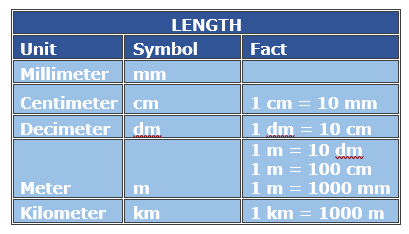# Metric Distance Conversion with Whole Number Values

Metric units are easy to work with, since they are all multiples of ten (or a hundred, or one-tenth, and so on) of each other. You can convert between the various units of different sizes by merely moving the decimal point the correct number of places.

The most common metric measurements for length are the millimeter, the centimeters, the meter, and the kilometer.

Millimeters are small units of length. Thickness of photo-id card, the thickness of a mobile phones and so on are of the order of millimeters. 10 such millimeters make a centimeter.

The length of a notebook, height of people, width of a table and so on can be measured in centimeters but the length of a basketball court is better measured in meters. 1 meter = 100 centimeters.

The length of a guitar, the height of a room, the length of a football field and so on are measured in meters.

The distance between places is similarly measured conveniently in kilometers. 1 kilometer = 1000 meters.

The tables below help in converting between these various metric units of length.If you have this Do this To get this
millimeters(mm) Divide by 10(mm/10) centimeter(cm)
centimeters(cm) Multiply by 10 (cm*10) Millimeters(cm)
Meters(m) Multiply by 100 (m*100) Centimeters(cm)
Centimeters(cm) Divide by 100(cm/100) Meters(m)
Millimeters(mm) Divide by 1000(mm/1000) Meters(m)

Fill in the blank to make the conversion true.

73 meters = ____centimeters

### Solution

Step 1:

1 meter = 100 cm

Step 2:

73 meters = 73 m × 100 = 7300 cm

So, 73 meters = 7300 cm

Fill in the blank to make the conversion true.

90 millimeters = ____centimeters

### Solution

Step 1:

10 millimeter = 1 cm; 1 mm = 1/10 cm

Step 2:

90 mm = 90 mm × 1/10 = 9 cm

So, 90 mm = 9 cm

Fill in the blank to make the conversion true.

300 centimeters = ____meters

### Solution

Step 1:

100 centimeter = 1 meter; 1 cm = 1/100 m

Step 2:

300 cm = 300 × 1/100 = 3 m

So, 300 cm = 3 m